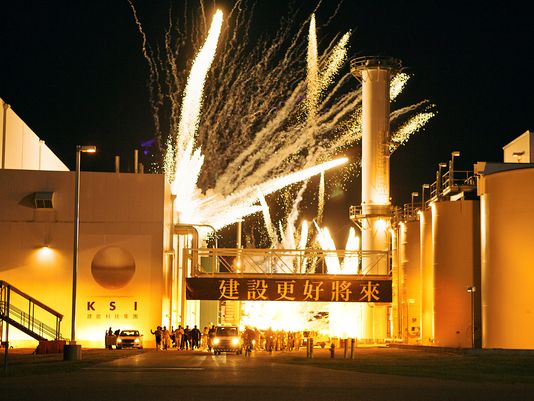# (Python) spawing scripts crashing blender 2.71

I’ve created 2 scripts:

``````#this script generates random numbers between 1 and 3 and spawns corresponding objects
#v1.0
#by me

import bge
import random

cont = bge.logic.getCurrentController()
scene = bge.logic.getCurrentScene()
own = cont.owner

move = cont.actuators['Motion']
number = own['number']

if number &gt;= 0:
own['number'] = (random.randint(1,3))
cont.activate(move)

if number == 1:
own['number'] = 0

if number == 2:
own['number'] = 0

if number == 3:
own['number'] = 0
``````

and this one:

``````#this script generates a random orientation to shoot off sparks in
#v1.0
#by me

import bge
import random

cont = bge.logic.getCurrentController()
scene = bge.logic.getCurrentScene()
own = cont.owner

#number of spark branches

branches = own['branches']

if branches &gt; 0:

RandRot = [(random.randint(0,180)), (random.randint(0,180)), (random.randint(0,180))];

own.applyRotation((RandRot),0)

own['branches'] += (-1)
``````

Playing the game (and exiting) exactly 10 times crashes blender (v2.71).
The console isn’t bringing up any errors either.

Basically I’m trying to replace a logic brick setup for a small spark explosion (and its worked so far), the main spawn shoots of spark spawners at a random direction, however as mentioned crashes blender after using it 10 times.

I was wondering if there is something that would cause this to happen?

and also if the code could be improved for efficiency?

.blend file here if you want to check it out

Thanks!

``````own['branches'] += (-1)
``````
``````own['branches'] -= 1
``````

Also I don’t understand the spawning of an spawner?
Do you want them to split after some (random) time?
That would be interesting and dangerous.

My simple take on this.

``````import bgeimport random

def main():

cont = bge.logic.getCurrentController()
own = cont.owner
scene = bge.logic.getCurrentScene()

spark_types = [ "Cube", "Cone", "Icosphere", "Suzanne", "Torus"]

if own['prop'] &gt; 0:
own['prop'] -= 1

selected = random.choice( spark_types)
v = 20
x,y,z = [ random.randint(-v,v) for i in range(3)]
spark.setLinearVelocity((x,y,abs(z) ))

main()
``````

### Attachments

spark.blend (606 KB)

if number >= 0:
own[‘number’] = (random.randint(1,3))
cont.activate(move)

if number == 1:
own[‘number’] = 0

if number == 2:
own[‘number’] = 0

if number == 3:
own[‘number’] = 0

I dont understand how this is layed out. Using variables with the exact same name as properties then changing the property to match the variable with the exact same name just to change the variable back again confuses me and gives me a headache to try to decipher. Maybe something is crashing the script out. your first condition is always going to be true if one of the others are true. Because 1 2 and 3 three are all >= 0, And then if the scrip sees that a number is not zero it sets it back to zero and starts over, so its trying to run multiple conditions constantly. Not sure if that would cause it to crash out, but you should clean that code up. Use some elifs. Wierd that it does it only after exatcly 10 times. The codes just so loopy that I cant understand exatcly what your trying to do to clean it up. Does it run everyframe?

Edit.
Vegetables code looks good and clean (although I dont understand why people always name their function main() I would name it sparkGenerator() or something, but people just do that). And random.choice(list name) is always nice, as it will pick items out of a list and you dont have to structure it with, If 1 is picked do this, if 2 is picked do this. You can go straight to object list that you create and let it pick one for you.

Thanks for the exampleAs shown in the .blend I want the moving ‘spawners’ to have streaks of sparks behind them. So instead of just one spark texture flying out, I want a trail being shot off at a random velocity.
something like this:obviously the detail will be limited but something close to this would be the end goal.

I used a random rotating empty to simulate a cluster of different empties that before with logic bricks would shoot off one spark trail each.

Yeah I agree, after some revision the first if statement probably isn’t needed with an always sensor on a true pulse joint to the script. Using an object list would probably also neaten up the code a little. Thanks for the suggestions, I’m fairly new to python so I still got a lot of learning to doOk, so after lots of trial and error I still haven’t been able to get anything working, the .blend started doing weird stuff like wiping animations upon crashing even if they were saved. So I thought that the .blend might be corrupted and appended it into another .blend however still got the same result using these scripts as well:

For the explosion spawner:

``````#this script spawns in a certain number of spark branches#v1.2
#by me

import bge
import random

def branches():

cont = bge.logic.getCurrentController()
scene = bge.logic.getCurrentScene()
own = cont.owner

if own['branches'] &gt; 0:
own['branches'] -= 1

spark = scene.addObject("spark branch", own, (random.randint(30, 60)))
vel = 12
x,y,z = [random.randint(-vel, vel) for i in range(3)]
#abs() to make the branches fly upwards
spark.setLinearVelocity((x,y, abs(z)))

branches()
``````

and for each individual spark stream:

``````#this script generates random sparks.#v1.2
#by me

import bge
import random

def RandomGen():

cont = bge.logic.getCurrentController()
scene = bge.logic.getCurrentScene()
own = cont.owner

Spark_Types = ['spark1', 'spark2', 'spark3']

Spark = random.choice(Spark_Types)

RandomGen()
``````

It might be the due to the multiple spawning of objects with individual spawning scripts but either way it seems crash blender.
Again I’m still learning python, but until I can figure out what I have done wrong I will stick to using logic bricks.

Thanks

Switch to 2.70 :yes:

Thanks, 2.70a works fine :D!

Not sure what exactly to report as a bug but hopefully this will be fixed by 2.72…